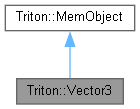Triton Visual simulation library for ocean rendering.
Triton::Vector3 Class Reference

A 3D double-precision Vector class and its operations. More...

`#include <Vector3.h>`

Inheritance diagram for Triton::Vector3:[legend]
Collaboration diagram for Triton::Vector3:[legend]

## Public Member Functions

Vector3 ()
Default constructor, initializes to (0,0,0). More...

Vector3 (double px, double py, double pz)
Constructs a Vector3 with the given x,y,z coordinates. More...

Vector3 (const double *p)
Constructs a Vector 3 from a pointer to 3 doubles.

Vector3 (const Vector3 &v)
Copy constructor.

double TRITONAPI Length () const
Returns the length of the vector. More...

double TRITONAPI SquaredLength () const
Returns the squared length of the vector, which is faster than computing the length. More...

void TRITONAPI Normalize ()
Scales the vector to be of length 1.0. More...

double TRITONAPI Dot (const Vector3 &v) const
Determines the dot product between this vector and another, and returns the result. More...

Vector3 TRITONAPI Cross (const Vector3 &v) const
Determines the cross product between this vector and another, and returns the result. More...

Vector3 TRITONAPI operator * (double n) const
Scales each x,y,z value of the vector by a constant n, and returns the result. More...

Vector3 TRITONAPI operator * (const Vector3 &v) const
Multiplies the components of two vectors together, and returns the result. More...

Vector3 TRITONAPI operator+ (double n) const
Adds a constant n to each component of the vector, and returns the result. More...

Vector3 TRITONAPI operator - (const Vector3 &v) const
Subtracts the specified vector from this vector, and returns the result. More...

Vector3 TRITONAPI operator+ (const Vector3 &v) const
Adds this vector to the specified vector, and returns the result. More...

bool TRITONAPI operator== (const Vector3 &v) const
Tests if two vectors are exactly equal. More...

bool TRITONAPI operator != (const Vector3 &v) const
Test if two vectors are not exactly equal. More...

void TRITONAPI Serialize (std::ostream &s) const
Write this vector's data to a file. More...

void TRITONAPI Unserialize (std::istream &s)
Restore this vector from a file. More...

## Public Attributes

double x
Data members x,y,z public for convenience. More...

## Detailed Description

A 3D double-precision Vector class and its operations.

## ◆ Vector3() [1/2]

 Triton::Vector3::Vector3 ( )
inline

Default constructor, initializes to (0,0,0).

## ◆ Vector3() [2/2]

 Triton::Vector3::Vector3 ( double px, double py, double pz )
inline

Constructs a Vector3 with the given x,y,z coordinates.

## ◆ Cross()

 Vector3 TRITONAPI Triton::Vector3::Cross ( const Vector3 & v ) const
inline

Determines the cross product between this vector and another, and returns the result.

## ◆ Dot()

 double TRITONAPI Triton::Vector3::Dot ( const Vector3 & v ) const
inline

Determines the dot product between this vector and another, and returns the result.

## ◆ Length()

 double TRITONAPI Triton::Vector3::Length ( ) const
inline

Returns the length of the vector.

## ◆ Normalize()

 void TRITONAPI Triton::Vector3::Normalize ( )
inline

Scales the vector to be of length 1.0.

## ◆ operator !=()

 bool TRITONAPI Triton::Vector3::operator != ( const Vector3 & v ) const
inline

Test if two vectors are not exactly equal.

## ◆ operator *() [1/2]

 Vector3 TRITONAPI Triton::Vector3::operator * ( double n ) const
inline

Scales each x,y,z value of the vector by a constant n, and returns the result.

## ◆ operator *() [2/2]

 Vector3 TRITONAPI Triton::Vector3::operator * ( const Vector3 & v ) const
inline

Multiplies the components of two vectors together, and returns the result.

## ◆ operator -()

 Vector3 TRITONAPI Triton::Vector3::operator - ( const Vector3 & v ) const
inline

Subtracts the specified vector from this vector, and returns the result.

## ◆ operator+() [1/2]

 Vector3 TRITONAPI Triton::Vector3::operator+ ( double n ) const
inline

Adds a constant n to each component of the vector, and returns the result.

## ◆ operator+() [2/2]

 Vector3 TRITONAPI Triton::Vector3::operator+ ( const Vector3 & v ) const
inline

Adds this vector to the specified vector, and returns the result.

## ◆ operator==()

 bool TRITONAPI Triton::Vector3::operator== ( const Vector3 & v ) const
inline

Tests if two vectors are exactly equal.

## ◆ Serialize()

 void TRITONAPI Triton::Vector3::Serialize ( std::ostream & s ) const
inline

Write this vector's data to a file.

## ◆ SquaredLength()

 double TRITONAPI Triton::Vector3::SquaredLength ( ) const
inline

Returns the squared length of the vector, which is faster than computing the length.

## ◆ Unserialize()

 void TRITONAPI Triton::Vector3::Unserialize ( std::istream & s )
inline

Restore this vector from a file.

## ◆ x

 double Triton::Vector3::x

Data members x,y,z public for convenience.

The documentation for this class was generated from the following file: